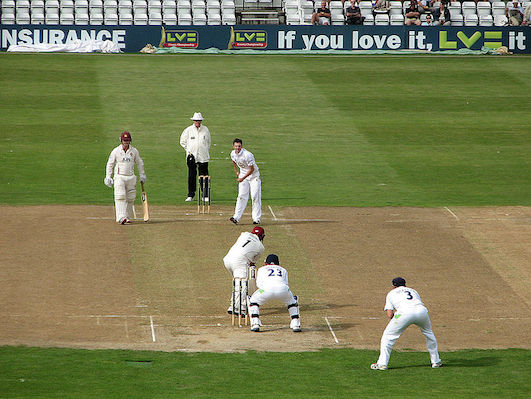# Let's play cricketThere is a hypothetical cricket match going on in kolkata between India vs Australia. Presently there is a spinner of height 2 metre bowling first ball of the over to a right hander.He is bowling a leg stump line, his ball deceives the batsmen and he gets bowled.

In the xyz,co-ordinate space consider the pitch to be the region $0 \le x \le 20, -2 \le y \le 2,z=0$

Consider stumps to be the lines

$0 \le z \le 0.5,x=20,y=0.125$

$0 \le z \le 0.5,x=20,y=0$

$0 \le z \le 0.5,x=20,y=-0.125$

The ball is released from the point $(0,-0.125,2)$ with a velocity $30 \hat{i}$ m/s, also given an angular velocity of $-\omega \hat{i}, \omega \ge 0$.

The question is simple, if the maximum value of $\omega$, for which the batsmen can get bowled is $=\frac { a(b+c\sqrt { b } ) }{ d }$ rad/s where $a,b,c,d$ are integers, $a,d$ are co-prime,$b$ is not divisible by any square prime number then find $a+b+c+d$.

Details and Assumptions

1) Assume ball a solid sphere of radius $0.01 m, g= 10 m{s}^{-2}$.Consider the pitch to be sufficiently rough and the collision to be elastic.

2) All the co-ordinates are given in metres.

3) If in the trajectory of the ball it is hitting the stumps, consider the batsmen bowled.

4) Consider it as a point mass while calculating it's trajectory

###### Image credit: Wikipedia John Sutton
×

Problem Loading...

Note Loading...

Set Loading...